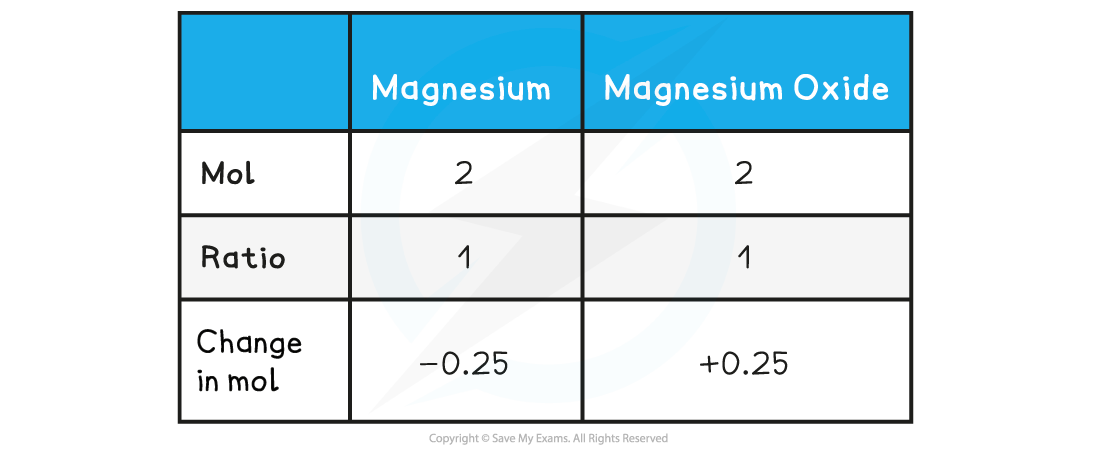# AQA A Level Chemistry复习笔记1.3.2 Reacting Masses

### Reacting Masses

• The number of moles of a substance can be found by using the following equation:• It is important to be clear about the type of particle you are referring to when dealing with moles
• E.g. 1 mole of CaF2 contains one mole of CaF2 formula units, but one mole of Ca2+ and two moles of F- ions

#### Reacting masses

• The masses of reactants are useful to determine how much of the reactants exactly react with each other to prevent waste
• To calculate the reacting masses, the chemical equation is required
• This equation shows the ratio of moles of all the reactants and products, also called the stoichiometry, of the equation
• To find the mass of products formed in a reaction the following pieces of information are needed:
• The mass of the reactants
• The molar mass of the reactants
• The balanced equation

#### Worked Example

Mass calculation using molesCalculate the mass of magnesium oxide that can be made by completely burning 6 g of magnesium in oxygen.

magnesium (s)  +  oxygen (g)  → magnesium oxide (s)

Step 1: The symbol equation is:

2Mg (s)    +     O2 (g)      →      2MgO (s)

Step 2: The relative formula masses are:

Magnesium : 24         Oxygen : 32         Magnesium Oxide : 40

Step 3: Calculate the moles of magnesium used in reactionStep 4: Find the ratio of magnesium to magnesium oxide using the balanced chemical equationTherefore, 0.25 mol of MgO is formed

Step 5: Find the mass of magnesium oxide

mass = mol x Mr

mass = 0.25 mol x 40 g mol-1mass = 10 g

Therefore, mass of magnesium oxide produced is 10 g

#### Stoichiometric relationships

• The stoichiometry of a reaction can be found if the exact amounts of reactants and products formed are known
• The amounts can be found by using the following equation:• The gas volumes can be used to deduce the stoichiometry of a reaction
• E.g. in the combustion of 50 cm3 of propane reacting with 250 cm3 of oxygen, 150 cm3  of carbon dioxide is formed suggesting that the ratio of propane:oxygen:carbon dioxide is 1:5:3

C3H8 (g) + 5O2 (g) → 3CO2 (g) + 4H2O (l)